### Chemical And Heat Effects Of Electric Currents

Chapter XIII. Chemical And Heat Effects Of ElectricCurrents.

THE CHEMICAL AND HEAT EFFECTS OF ELECTRIC CURRENTS

### (1) The Chemical Effect of an Electric Current

280. Electroplating.?If two carbon rods (electric light carbons answer very well) are placed in a solution of copper sulphate (Fig. 260) and then connected by wires to the binding posts of an electric battery, one of the rods soon becomes covered with a coating of metallic copper while bubbles of gas may be seen upon the other carbon. If a solution of lead acetate is used in the same way a deposit of metallic lead is secured, while a solution of silver nitrate gives silver.Fig. 260.?Two carbons placed in a solution of copper sulphate.
Fig. 261.?An electroplating bath.
This process of depositing metals upon the surface of solids by an electric current is called electroplating. Everyone has seen electroplated articles such as silver plated knives, forks, and spoons, and nickel-plated rods, handles, etc. Copper electrotype plates such as are used in printing school books are made by this process. In[Pg 308] practical electroplating a solution of the metal to be deposited is placed in a tank; across the top of this tank are placed copper rods to act as conducting supports. From one of these rods, the cathode, objects to be plated are hung so as to be immersed in the liquid. From other rods, the anodes, are hung plates of the metal to be deposited. These are dissolved as the current deposits a coating upon the articles, thus keeping the solution up to its proper strength. (See Fig. 261.)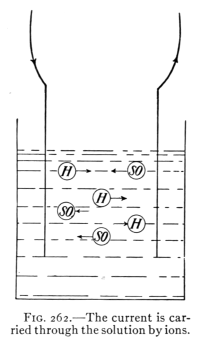Fig. 262.?The current is carried through the solution by ions.
281. Electrolysis.?A solution from which a deposit is made by an electric current is called an electrolyte. The plates or other objects by which the current enters or leaves the electrolyte are called the electrodes. The electrode by which the current enters is called the anode (an = in) while the electrode by which it leaves is the cathode (cath = away). The process by which an electric current decomposes a solution and deposits a substance upon an electrode is called electrolysis. The current always flows within the cell from anode to the cathode. (See Fig. 262.) The metal goes with the current and is found deposited upon the cathode.
282. Theory of Electrolysis.?The action going on in an electrolytic cell has been carefully studied. The theory of electrolysis, which is supported by much experimental evidence, supposes that many of the molecules in a dilute solution of a substance "split up" into two parts called[Pg 309] "ions," one ion having a positive, the other a negative charge. In a dilute solution of sulphuric acid, the positive ion is of hydrogen, while the negative ion is the (SO4) or sulphion. These ions bearing electric charges are believed to be the carriers of the electric current through the electrolyte.
The positive ions move with the current from the anode to the cathode, while the negative ions apparently are repelled by the cathode and appear upon the anode. Evidence of the accumulation of the two kinds of ions at the two electrodes is furnished by the electrolysis of water, described below.
283. Electrolysis of Water.?Two glass tubes (Fig. 263), H and O, are attached at the bottom to a horizontal glass tube. To the latter is also connected an upright tube T. At the lower ends of H and O are inserted, fused in the glass, platinum wires, A and C. The tubes are filled with a weak solution of sulphuric acid. The tops of H and O are closed with stopcocks, T being open; a current of electricity is sent in at A and out at C. A movement of the ions at once begins, the positive hydrogen ions appearing at C. These accumulate as bubbles of hydrogen which rise to the top of H and displace the liquid. At the same time bubbles of oxygen appear at A. These rise in O and also displace the liquid which rises in T. After the action has continued some time it may be noticed that the volume of hydrogen is just twice that of the oxygen. This was[Pg 310] to have been expected since the formula for water is H2O. The nature of the gas in H or O may be tested by opening the stopcock and allowing the gas to escape slowly. The hydrogen gas can be lighted by a flame while the oxygen gas will cause a spark upon a piece of wood to glow brightly, but does not burn itself.Fig. 263.-Electrolysis of water; oxygen collects in O, hydrogen in H.
284. Evidence that ions are necessary to conduct a current in a liquid is furnished by the following experiment. A quart jar is carefully cleaned, and half filled with distilled water. Two pieces of zinc 5 cm. square are soldered to pieces of rubber-insulated No. 14 copper wire. The zincs are placed in the distilled water (Fig. 264) and the wires are connected to a 110 volt circuit with a 16 candle-power incandescent lamp in series with the cell, as in the figure. If the zincs have been carefully cleansed and the water is pure, no current flows as is shown by the lamp remaining dark. If a minute quantity of sulphuric acid or of common salt is placed in the water the lamp at once begins to glow. Ions are now present in the liquid and conduct the current. That some substances in solution do not form ions may be shown by adding to another jar of pure water some glycerine and some cane sugar, substances resembling the acid and salt in external appearance but which do not ionize when dissolved as is shown by the lamp remaining dark after adding the glycerine and sugar. The acid and salt are of mineral origin while the glycerine and sugar are vegetable products. This experiment illustrates the principle that the water will conduct only when it contains ions.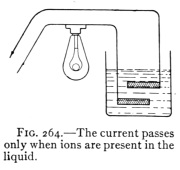Fig. 264.?The current passes only when ions are present in the liquid.
[Pg 311]
285. Laws of Electrolysis.?These were discovered by Faraday in 1833, and may be stated as follows: I. The mass of a substance deposited by an electric current from an electrolyte is proportional to the intensity of current which passes through it.
II. The mass of any substance deposited by a current of uniform intensity is directly proportional to the time the current flows.
These laws have been used as a basis for defining and measuring the unit of current flow, the ampere. (See Art. 264.)
286. Instances of Electrolysis.?(a) Medicines, especially those containing a mineral substance, are sometimes introduced into the human body by electrolysis. (b) Water and gas pipes are sometimes much weakened by the effects of electric currents in the earth, especially return currents from street railways. Such currents use the metal pipes as a conductor. At the place where the current leaves the metal and enters the ground, it removes metallic ions from the pipe. This process continuing, the pipe becomes weakened and at length breaks. (c) Copper is purified by the use of electric currents that remove the copper from ore or from other metals and deposit it upon electrodes. Electrolytic copper is the purest known. (d) Aluminum is obtained by the use of large currents of electricity that first heat the material used until it melts and then deposit the metal from the fluid material by electrolysis. These results are called chemical effects of the current since by the use of electric currents substances are changed chemically, that is, they are separated into different chemical substances.
[Pg 312]

#### Important Topics

1. Electrolysis, electroplating, anode, cathode, ion.
2. Theory of electrolysis?evidence: (a) electrolysis of water; (b) conductivity of acid and water.
3. Laws of electrolysis.
4. Practical use of electrolysis.

#### Exercises

1. A dynamo has an E.M.F. of 10 volts. What is the resistance in the circuit when 20 amperes are flowing?
2. How much silver will be deposited in an hour by this current?
3. Name five objects outside of the laboratory that have been acted upon by electrolysis. How in each case?
4. Why is table ware silver plated? Why are many iron objects nickel plated?
5. How is the electrolysis of water pipes prevented?
6. Two grams of silver are to be deposited on a spoon by a current of 1 ampere. Find the time required.
7. How long will it take to deposit 20 g. of silver in an electroplating bath if a current of 20 amperes is used?
8. If 1000 g. of silver are deposited on the cathode of an electrolytic reduction plant in 10 minutes, what is the current intensity employed?

### (2) The Storage Battery and Electric Power

287. Differences Between Voltaic and Storage Cells. Voltaic cells in which electric currents are produced by the chemical action between metal plates and an electrolyte are often called primary batteries. In voltaic cells one or both plates and the electrolyte are used up or lose their chemical energy in producing the current and after a time need to be replaced by new material, the chemical energy of the electrolyte and of one of the plates having been transformed into electrical energy.
A different proceeding obtains with another type of[Pg 313] cell. This is called a storage battery, or an accumulator. In these cells, the same plates and electrolyte are used without change for extended periods, sometimes for a number of years. For this reason storage batteries have displaced many other types of cells, and they are now used (a) to operate many telephone, telegraph, and fire-alarm circuits, (b) to work the spark coils of gas and gasoline engines, (c) to help carry the "peak" load upon lighting and power circuits and (d) to furnish power for electric automobiles. Since a storage battery can deliver an electric current only after an electric current from an outside source has first been sent through it, they are often called secondary batteries.
288. Construction and Action of a Storage Cell.?The common type of storage cells consists of a number of perforated plates made of an alloy of lead and a little antimony. (See Figs. 265, 266, 267.) Into the perforations is pressed a paste of red lead and litharge mixed with sulphuric acid. The plates are placed in a strong solution (20 to 25 per cent.) of sulphuric acid. The plates are now ready to be charged. This is accomplished by sending a direct current from an electric generator through the cell. The hydrogen ions are moved by the current to one set of plates and change the paste to spongy metallic lead. The sulphions move to the other set of plates and change the paste to lead oxide. This electrolytic action causes the two plates to become quite different chemically so that when the cell is fully charged it is like a voltaic cell, in having plates that are different chemically. It has, when fully charged, an E.M.F. of about 2.2 volts. The several plates of a cell being in parallel and close together, the cell has but small internal resistance. Consequently a large current is available.Fig. 265.?The positive plate of a storage cell.
Fig. 266.?The negative plate of a storage cell.Fig. 267.?A complete storage cell.
Fig. 268.
About 75 per cent. of the energy put into the storage cell[Pg 314] in charging can be obtained upon discharging. Therefore the efficiency of a good storage cell is about 75 per cent. Fig. 268 represents a storage battery connected to charging and discharging circuits. The lower is the charging circuit. It contains a dynamo and a resistance (neither of which are shown in the figure) to control the current sent into the cell. The charging current enters the positive pole and leaves by the negative pole. The current produced by the cell, however, flows in the opposite direction through it, that is, out from the positive and in at the negative pole. This current may be controlled by a suitable resistance and measured by an ammeter. Storage cells have several advantages: (a) They can be charged and discharged a great many times before the material placed in the perforations in the plates falls out.[Pg 315] (b) The electrical energy used in charging the plates costs less than the plates and electrolyte of voltaic cells. (c) Charging storage cells takes much less labor than replacing the electrolyte and plates of voltaic cells. (d) Storage cells produce larger currents than voltaic cells. The two principal disadvantages of storage cells are that (a) they are very heavy, and (b) their initial cost is considerable.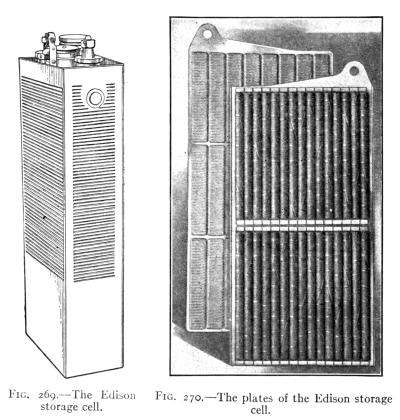Fig. 269.?The Edison storage cell.
Fig. 270.?The plates of the Edison storage cell.
289. The Edison storage cell (Figs. 269 and 270) has plates of iron and nickel oxide. The electrolyte is a strong solution of potassium hydroxide. These cells[Pg 316] are lighter than lead cells of the same capacity and they are claimed to have a longer life.
290. Energy and Power of a Storage Cell.?In a storage cell, the electrical energy of the charging current is transformed into chemical energy by the action of electrolysis. It is this chemical energy that is transformed into the energy of the electric current when the cell is discharged. The capacity of storage cells is rated in "ampere hours," a 40 ampere hour cell being capable of producing a current of 1 ampere for 40 hours, or 5 amperes for 8 hours, etc. The production and extensive use of electric currents have made necessary accurate methods for measuring the energy and power of these currents. To illustrate how this is accomplished, let us imagine an electric circuit as represented in Fig. 268. Here four storage cells in series have an E.M.F. of 8 volts and in accordance with Ohm's law produce a current of 2 amperes through a resistance in the circuit of 4 ohms. Now the work done or energy expended by the current in passing through the resistance between the points M and N depends upon three factors (1) the E.M.F. or potential difference; (2) the current intensity and (3) the time. The energy is measured by their product. That is, electrical energy = potential difference ? current intensity ? time. This represents the electrical energy in joules, or
Joules = volts ? amperes ? seconds, or
j = E ? I ? t.

In the circuit represented in Fig. 268 the energy expended between the points M and N in 1 minute (60 seconds) is 8 ? 2 ? 60 = 960 joules.
291. Electric Power.?Since power refers to the time rate at which work is done or energy expended, it may be computed by dividing the electrical energy by the time,[Pg 317] or the electrical power = volts ? amperes. The power of 1 joule per second is called a watt. Therefore,
Watts = volts ? amperes, or
Watts = E ? I.

Other units of power are the kilowatt = 1000 watts and the horse-power = 746 watts. In the example given in Art. 290 the power of the current is 8 ? 2 = 16 watts, or if the energy of the current expended between the joints M and N were converted into mechanical horse-power it would equal 16/746 of a horse-power. Electrical energy is usually sold by the kilowatt-hour, or the amount of electrical energy that would exert a power of 1000 watts for one hour, or of 100 watts for 10 hours, or of 50 watts for 20 hours, etc.

#### Important Topics

2. Electric energy, unit value, how computed?
3. Electric power, three units, value, how computed, how sold?

#### Exercises

1. In what three respects are voltaic and storage cells alike? In what two ways different?
2. Name the four advantages of storage cells in the order of their importance. Give your reasons for choosing this order.
3. Why are dry cells more suitable for operating a door-bell circuit, than a storage battery? Give two reasons.
4. The current for a city telephone system is provided by a storage battery. Why is this better than dry cells at each telephone?
5. An incandescent lamp takes 0.5 ampere at 110 volts. What power is required to operate it? How much energy will it transform in 1 minute?
6. How long would it take for this lamp to use a kilowatt hour of energy?
7. A street car used 100 amperes at 600 volts pressure. What[Pg 318] power was delivered to it? Express also in kilowatts and horse-power.
8. An electric toaster takes 5 amperes at 110 volts. If it toasts a slice of bread in 2 minutes, what is the cost at 10 cents a kilowatt hour?
9. An electric flat iron takes 5 amperes at 110 volts. Find the cost of using it for 2 hours at 12 cents a kilowatt hour.
10. A 1/4 kilowatt motor is used to run a washing-machine for 5 hours. What is the expense for this power at 10 cents a kilowatt hour?
11. What is the efficiency of a motor that takes 7390 watts and develops 9 horse-power?
12. How many horse-power are there in a water-fall 212 ft. high over which flows 800 cu. ft. of water per second? Express this power in kilowatts.
13. What horse-power must be applied to a dynamo having an efficiency of go per cent. if it is to light 20 arc lamps in series, each taking 10 amperes at 60 volts?

### (3) The Heat Effect of Electric Currents

292. The Production of Heat by an Electric Current.?When no chemical or mechanical work is done by an electric current its energy is employed in overcoming the resistance of the conducting circuit and is transformed into heat. This effect has many practical applications and some disadvantages. Many devices employ the heating effect of electric currents, (a) the electric furnace, (b) electric lights, (c) heating coils for street cars, (d) devices about the home, as flat irons, toasters, etc. Sometimes the heat produced by an electric current in the wires of a device such as a transformer is so large in amount that especial means of cooling are employed. Unusually heavy currents have been known to melt the conducting wires of circuits and electrical devices. Hence all circuits for electric power as well as many others that ordinarily carry small currents are protected by fuses. An electric fuse[Pg 319] is a short piece of wire that will melt and break the circuit if the current exceeds a determined value. The fuse wire is usually enclosed in an incombustible holder. Fuse wire is frequently made of lead or of an alloy of lead and other easily fusible metals. (See Figs. 271 and 272.)Fig. 271.?A type of enclosed fuse.
Fig. 272.?A link fuse (above); plug fuses (below).
293. Heat Developed in a Conductor.?A rule for computing the amount of heat produced in an electric circuit by a given current has been accurately determined by experiment. It has been found that 1 calorie of heat (Art. 142), is produced by an expenditure of 4.2 joules of electrical (or other) energy. In other words, 1 joule will produce 1/4.2 or 0.24 calorie. Now the number of joules of electrical energy in an electric circuit is expressed by the following formula:
Joules = volts ? amperes ? seconds, or since 1 joule = 0.24 calorie,
Calories = volts ? amperes ? seconds ? 0.24 or
H = EI ? t ? 0.24 (1)

By Ohm's law, I = E/R or E = I ? R, substituting in equation (1) IR for its equal E we have
H = I2R ? t ? 0.24 (2)

Also since I = E/R substitute E/R for I in equation (1) and we have
H = E2/R t ? 0.24 (3)

[Pg 320]
To illustrate the use of these formulas by a problem suppose that a current of 10 amperes is flowing in a circuit having a resistance of 11 ohms, for 1 minute. The heat produced will be by formula (2) = (10)2 ? 11 ? 60 ? 0.24 equals 15,840 calories.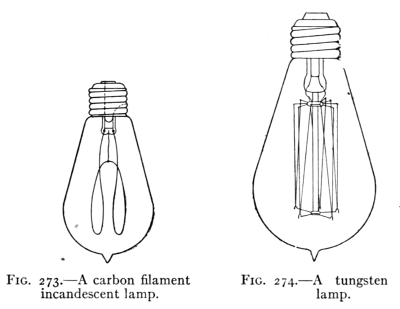Fig. 273.?A carbon filament incandescent lamp.
Fig. 274.?A tungsten lamp.
294. The Incandescent Lamp.?One of the most common devices employing the heat effect of an electric current is the incandescent lamp. (See Fig. 273.) In this lamp the current is sent through a carbon filament, which is heated to incandescence. In order to keep the filament from burning as well as to prevent loss of heat by convection, it is placed in a glass bulb from which the air is exhausted. Two platinum wires fused in the glass connect the carbon filament with the grooved rim and the end piece of the base. The end piece and rim connect with the socket so that an electric current may flow through the filament of the lamp. The carbon incandescent lamp has a low efficiency. It takes 0.5 ampere of current at 110 volts or in other words it requires 55 watts to cause a 16-candle-power[Pg 321] lamp to glow brightly, hence 1 candle power in this lamp takes 55/16 = 3.43 watts.
The efficiency of electric lamps is measured by the number of watts per candle power. This is a peculiar use of the term efficiency, as the larger the number the less efficient is the lamp. More efficient lamps have been devised with filaments of the metals tantalum and tungsten (Fig. 274). These give a whiter light than do carbon lamps, and consume but about 1.25 watts per candle power.
Comparative "Efficiency" of Electric Lamps
 Name of lamp Watts per candle power Name of lamp Watts per candle power Carbon filament 3 to 4 Arc lamp 0.5 to 0.8 Metallized carbon 2.5 Mercury arc 0.6 Tantalum 2.0 Flaming arc 0.4 Tungsten 1.0 to 1.5 Nitrogen-filled tungsten 0.6 to 0.7
Incandescent lamps are connected in parallel (see Fig. 254) to wires that are kept at a constant difference of potential of 110 or 115 volts. It is customary to place not more than twelve lamps upon one circuit, each circuit being protected by a fuse and controlled by one or more switches.
295. The Arc Light.?The electric arc light (see Fig. 275) is extensively used for lighting large rooms, also in stereopticons and motion picture machines. The light is intense, varying from 500 to 1700 candle power. The so-called mean spherical candle power of the arc light is about 510. The candle power in the direction of greatest intensity is about 1200. It is produced at an expenditure of[Pg 322] about 500 watts. It is therefore more efficient than the incandescent lamp, often taking less than 0.5 watt per candle power produced. The arc light was first devised by Sir Humphrey Davy in 1809, who used two pieces of charcoal connected to 2000 voltaic cells. The arc light requires so much power that its production by voltaic cells is very expensive. Consequently it did not come into common use until the dynamo had been perfected. Fig. 276 shows the appearance of the two carbons in an arc light. If a direct current is used the positive carbon is heated more intensely, and gives out the greater part of the light. The positive carbon is consumed about twice as fast as the negative and its end is concave, the negative remaining pointed.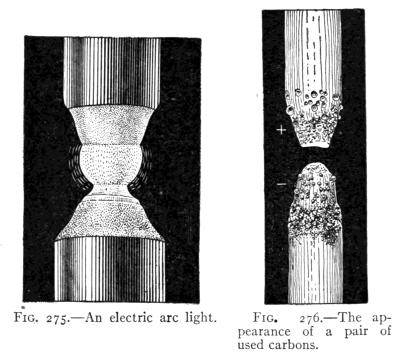Fig. 275.?An electric arc light.
Fig. 276.?The appearance of a pair of used carbons.
With alternating currents, the rods are equally consumed and produce equal amounts of light. In the stereopticon, the carbons are usually placed at right angles as[Pg 323] in Fig. 277. In the stereopticon as well as in outdoor lighting the direct current is more effective, although the alternating current is often used, since the latter can be produced and distributed more cheaply than can direct currents. In arc lamps, placing an inner glass globe (Fig. 278) about the carbons, decreases the consumption of the carbons materially. The carbon rods of enclosed arc lamps often last 60 to 100 hours.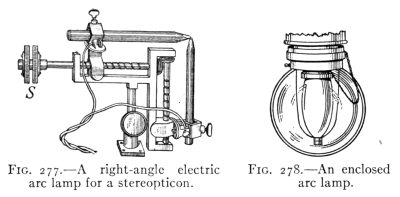Fig. 277.?A right-angle electric arc lamp for a stereopticon.
Fig. 278.?An enclosed arc lamp.
The reason why an open arc lamp needs to be "retrimmed" oftener than the enclosed lamp, that is, have new carbons placed in it, is because the carbons "burn" freely, that is unite with the oxygen of the air. In the enclosed arc lamp, the supply of oxygen in the inner globe is limited and is soon consumed, therefore the carbons last many times longer in such lamps.
Some carbon rods have soft cores containing calcium salts. These vaporize in the arc producing the flaming arc light of a bright yellow color, and give more light than the ordinary lamp.

#### Important Topics

1. Heat effects of electric currents, uses and applications.
2. Computation of the heat developed in a circuit. Three formulas.
3. Electric lamps; incandescent and arc; construction, uses, efficiency.
[Pg 324]

#### Exercises

1. Sketch a circuit containing 10 incandescent lamps in parallel. If each lamp when hot has a resistance of 220 ohms, and the E.M.F. is 100 volts, what current will flow?
2. What will it cost to use these lights for 3 hours a day for 30 days at 10 cents a kilowatt hour?
3. How much heat will these lamps produce per minute?
4. How could you connect 110-volt lamps to a street car circuit of 660 volts? Explain this arrangement and draw a diagram.
5. A certain arc lamp required 10 amperes of current at 45 volts pressure. What would it cost at 10 cents per kilowatt hour if used 3 hours a day for 30 days?
6. Show a diagram of 3 arc lamps in series. If each takes 45 volts and 10 amperes, how much E.M.F. and current will they require?
7. If an electric toaster uses 5 amperes at 115 volts, how much heat will this develop in half an hour?
9. How much heat is developed in an electric toaster in 2 minutes, if it uses 5 amperes at 100 volts?
10. How many B.t.u.'s are given off in an electric oven that takes 10 amperes at 110 volts for 1 hour? (1 B.t.u. equals 252 calories.)
11. An electric heater supplies heat at the rate of 700 B.t.u.'s an hour. How much power does it require?
12. How many watts are required to operate 120 incandescent lamps in parallel if each takes 0.5 amperes at 110 volts?
13. An electric lamp takes 12 amperes at a P.D. of 110 volts. How many B.t.u.'s are radiated from it each second? How many calories?
14. If a 110-volt incandescent lamp is submerged for 10 minutes in 400 gr. of cold water while a current of 0.5 amperes is flowing, how many degrees centigrade will the water be warmed?
15. In an electric furnace a current of 3000 amperes is used at a P.D. of 10 volts. Find the heat developed in 1 minute.
16. How many candle power should a 20-watt tungsten lamp give if its efficiency is one watt per candle power?
17. What is the "efficiency" of a 40-watt tungsten lamp if it gives 34 candle power?
[Pg 325]

#### Review Outline: Current Electricity

Produced by?Chemical action; voltaic and storage cells.
 Three Principal Effects: Magnetic, electromagnet, uses and applications. Chemical, electrolysis, applications. Heat, lighting and heating devices.
Theories: (a) of voltaic cells, (b) of electrolysis.
Units: Ampere, ohm, volt, watt, joule, kilowatt, horse power.
Measurement:
• (a) magnetic effect; galvanometer, ammeter, voltmeter, wattmeter, Wheatstone bridge, construction and use.
• (b) chemical effect; voltameter.
Laws:
• (a) Right hand rules, for conductor and helix.
• (b) Resistance, Conductors in series and parallel.
• (c) Ohm's law, heat law, power law, 3 forms for each.
• (d) Cells in parallel and series.
Problems: Upon applications of the laws and formulas studied.
 Devices and Instruments: Voltaic cells; wet, dry, and Daniell. Electrolysis and the storage battery. Measuring instruments, electric bell, sounder, Heating and lighting devices.
Terms: Anode, cathode, electrolyte, ion, circuit switch, current, e.m.f., resistance, potential.